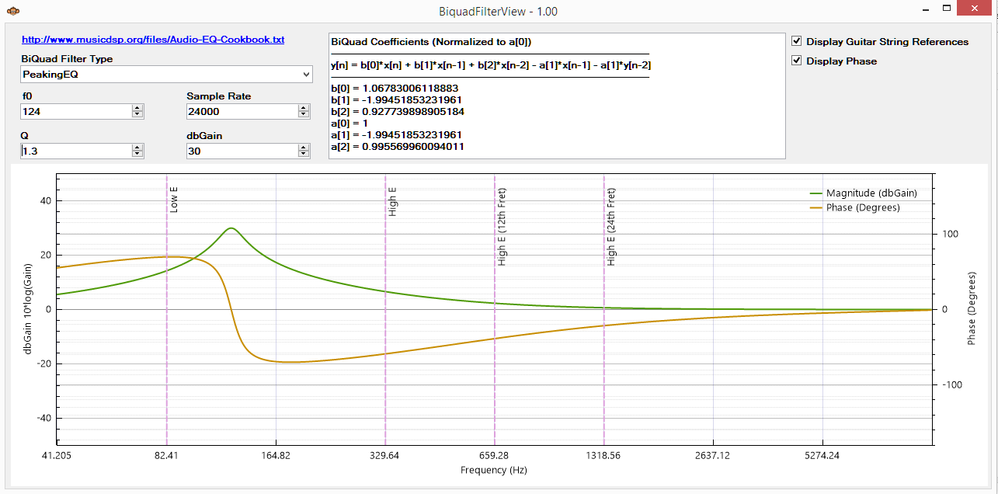Biquad Filter View - A IIR Biquad Filter Design & Visualization Tool

cancel
Showing results for
Did you mean:

No ratings

Summary:This tool is based upon the Audio BiQuad Cookbook Here:

http://www.musicdsp.org/files/Audio-EQ-Cookbook.txt

Very useful for configuring the FRDM-JAM and the MonkeyJam software!Requirements:

You need a machine with the .net 4.0 Framework (or greater) Installed (Windows 7 or Greater).    If you have issues go here:

Instructions:

Just unzip and run the .exe

Original Attachment has been moved to: BiQuadFilterView---1.0.0.zip

Tags (5)Hello Eli!

Thanks for this and other wonderful contributions to the community!

I am trying to implement CMSIS - Biquad Cascade IIR Filters Using Direct Form I Structure in my code and I am trying to calculate the coefficients using your tool. In the "http://www.musicdsp.org/files/Audio-EQ-Cookbook.txt" file, the equation is as follows:

y[n] = (b0/a0)*x[n] + (b1/a0)*x[n-1] + (b2/a0)*x[n-2] - (a1/a0)*y[n-1] - (a2/a0)*y[n-2]

However, in the BiQuadFilterView program of yours, in the info area, I can see that the equation is as follows:

y[n] = b*x[n] + b*x[n-1] + b*x[n-2] - a*x[n-1] - a*y[n-2]

In the Keil CMSIS documentation, the equation is as follows:

y[n] = b0 * x[n] + b1 * x[n-1] + b2 * x[n-2] + a1 * y[n-1] + a2 * y[n-2]

Notice the difference in between -a*x[n-1] - a*y[n-2] and + a1 * y[n-1] + a2 * y[n-2].

Is this a typo or the BiQuadFilterView uses a different algorithm?Hello Abdullah.

Sorry for the confusion.    Here are some pointers.

1.)

Equations 1 and 2 are really the same equation.    my b is just b/a in the 1st equation.    I keep the coefficients normalized to the a0 coefficient from the start.

You can get the source of the program here:

the file eDSP.cs has a function called "DesignBiquad" that shows how I get from one to the other.

2.)   Good observation on the keil implementation.   If you go through their documentation

They mention that they use a different sign convention for a1 and a2.   If you use BiQuadFilterView,  you will need to flip the sign.

3.)    Check out this project:

Freescale Hack It Together (H.I.T.) Project Initiative - Get Excited!

I didn't use the CMSIS implementation as it is optimized for block processing and I wanted per sample processing.   You can find an IIR implementation that implements some functions that compute fixed point coefficients for the biquad in C.

Good Luck!Hi Eli,

How do you scale the coefficients in the tool to -.99999 to +1 for Q15 format?

Many of the coefficients such as b and a are -1.99999 which is not Q15 compatible.

a is always 1.

Can I simply define a =2 and then scale the coefficients by 1/2?

Also, the dbGain setting seems to have no effect on the coefficients.

Thanks,